• MATLAB 进行gabor处理的之前，先对图片进行恢复处理
• 基于MATLAB图像恢复的环氧沥青混凝土断面处理研究.pdf
• 基于MATLAB图像恢复的环氧沥青混凝土断面处理研究
• '模糊和噪声图像' ); subplot( 222 );imshow(deconvwnr(BlurredNoisy,PSF,NSR),[]); title( 'deconbwnr(A,PSF,NSR)' ); subplot( 223 );imshow(deconvwnr(BlurredNoisy,PSF,NCORR,ICORR),[]); title( 'deconbwnr(A,...
目录

目录 1维纳滤波复原2规则化滤波复原程序3Lucy-Richardson复原程序举例4盲去卷积复原

1．维纳滤波复原
I=checkerboard(8);
noise=0.1*randn(size(I));
PSF=fspecial('motion',21,11);
Blurred=imfilter(I,PSF,'circular');
BlurredNoisy=im2uint8(Blurred+noise);
NP=abs(fftn(noise)).^2;
NPOW=sum(NP(:)/prod(size(noise));
NCORR=fftshift(real(ifftn(NP)));
IP=abs(fftn(I)).^2;
IPOW=sum(IP(:)/prod(size(noise));
ICORR=fftshift(real(ifftn(IP)));
ICORR1=ICORR(:,ceil(size(I,1)/2));
NSR=NPOW/IPOW;
subplot(221);imshow(BlurredNoisy,[]);
title('模糊和噪声图像');
subplot(222);imshow(deconvwnr(BlurredNoisy,PSF,NSR),[]);
title('deconbwnr(A,PSF,NSR)');
subplot(223);imshow(deconvwnr(BlurredNoisy,PSF,NCORR,ICORR),[]);
title('deconbwnr(A,PSF,NCORR,ICORR)');
subplot(224);imshow(deconvwnr(BlurredNoisy,PSF,NPOW,ICORR1),[]);
title('deconbwnr(A,PSF,NPOW,ICORR_1_D)');

2．规则化滤波复原程序
I=checkerboard(8);
PSF=fspecial('gaussian',7,10);
V=.01;
BlurredNoisy=imnoise(imfilter(I,PSF),'gaussian',0,V);
NOISEPOWER=V*prod(size(I));
[J LAGRA]=deconvreg(BlurredNoisy,PSF,NOISEPOWER);
subplot(221);imshow(BlurredNoisy);
title('A=Blurred and Noisy');
subplot(222);imshow(J);
title('[J LAGRA]=deconvreg(A,PSF,NP)');
subplot(223);imshow(deconvreg(BlurredNoisy,PSF,[],LAGRA/10));
title('deconvreg(A,PSF,[],0.1*LAGRA)');
subplot(225);imshow(deconvreg(BlurredNoisy,PSF,[],LAGRA/10))
subplot(224);imshow(deconvreg(BlurredNoisy,PSF,[],LAGRA*10));
title('deconvreg(A,PSF,[],10*LAGRA');
3．Lucy-Richardson复原程序举例
%Lucy-Richardson复原程序
I=checkerboard(8);
PSF=fspecial('gaussian',7,10);
V=.0001;
BlurredNoisy=imnoise(imfilter(I,PSF),'gaussian',0,V);
WT=zeros(size(I));
WT(5:end-4,5:end-4)=1;
J1=deconvlucy(BlurredNoisy,PSF);
J2=deconvlucy(BlurredNoisy,PSF,20,sqrt(V));
J3=deconvlucy(BlurredNoisy,PSF,20,sqrt(V),[],WT);
subplot(221);imshow(BlurredNoisy);
title('A=Blurred and Noisy');
subplot(222);imshow(J1);
title('deconvlucy(A,PSF)');
subplot(223);imshow(J2);
title('deconvlucy(A,PSF,NI,DP)');
subplot(224);imshow(J3);
title('deconvlucy(A,PSF,NI,DP,[],WT)');
4．盲去卷积复原
%盲去卷积复原
I=checkerboard(8);
PSF=fspecial('gaussian',7,10);
V=.0001;
BlurredNoisy=imnoise(imfilter(I,PSF),'gaussian',0,V);
WT=zeros(size(I));
WT(5:end-4,5:end-4)=1;
INITPSF=ones(size(PSF));
FUN=inline('PSF+P1','PSF','P1');
[J P]=deconvblind(BlurredNoisy,INITPSF,20,10*sqrt(V),WT,FUN,0);
subplot(221);imshow(BlurredNoisy);
title('A=Blurred and Noisy');
subplot(222);imshow(PSF,[]);
title('True PSF');
subplot(223);imshow(J);
title('Deblured Image');
subplot(224);imshow(P,[]);
title('Recovered PSF');

展开全文• 三、实验设备：安装有MATLAB 软件的计算机 四、实验原理 一幅退化的图像可以近似地用方程g=Hf+n 表示，其中g 为图像，H为变形算子，又称为点扩散函数（PSF ），f 为原始的真实图像，n 为附加噪声，它在图像捕获过程...


一、实验类型：验证性实验
二、实验目的
1. 掌握退化模型的建立方法。
2. 掌握图像恢复的基本原理。
三、实验设备：安装有MATLAB 软件的计算机
四、实验原理
一幅退化的图像可以近似地用方程g=Hf+n 表示，其中g 为图像，H为变形算子，又称为点扩散函数（PSF ），f 为原始的真实图像，n 为附加噪声，它在图像捕获过程中产生并且使图像质量变坏。其中，PSF 是一个很重要的因素，它的值直接影响到恢复后图像的质量。
I=I(60+[1:256],222+[1:256],:);
figure;imshow(I);
LEN=31;
THETA=11;
PSF=fspecial(‘motion’,LEN,THETA);
Blurred=imfilter(I,PSF,’circular’,’conv’);
figure;imshow(Blurred);
MATLAB 工具箱中有4 个图像恢复函数，如表3-1 所示。这4 个函数都以一个PSF 和模糊图像作为主要变量。deconvwnr 函数使用维纳滤波对图像恢复，求取最小二乘解，deconvreg 函数实现约束去卷积，求取有约束的最小二乘解，可以设置对输出图像的约束。deconvlucy 函数实现了一个加速衰减的Lucy-Richardson 算法。该函数采用优化技术和泊松统计量进行多次迭代。使用该函数，不需要提供有关模糊图像中附加噪声的信息。 deconvblind 函数使用的是盲去卷积算法，它在不知道PSF 的情况下进行恢复。调用deconvblind 函数时，将PSF 的初值作为一个变量进行传递。该函数除了返回一个修复后的图像以外，还返回一个修复后的PSF。下面以维纳滤波和约束去卷积为例说明图像恢复的实验原理。
deconvwnr使用维纳滤波对图像恢复deconvreg对图像进行约束去卷积deconvlucy用Lucy-Richardson 算法实现图像恢复deconvblind用盲去卷积算法实现图像恢复
1. 维纳滤波
使用deconvwnr 函数可以利用维纳滤波方法恢复图像。在图像的频率特征和附加噪声已知的情况下，Wiener 滤波比较有效。本例演示了维纳滤波器的性能，同时也演示了PSF 的重要性。得到准确的PSF 时，恢复的结果会比较好。
I=I(10+[1:256],222+[1:256],:);
LEN=31;
THETA=11;
PSF=fspecial(‘motion’,LEN,THETA);
Blurred=imfilter(I,PSF,’circular’,’conv’);
wnrl=deconvwnr(Blurred,PSF);
imshow(wnrl);
2. 约束去卷积
采用deconvreg 函数可以对图像进行约束去卷积。当知道附加噪声的部分信息时，使用约束去卷积实现图像恢复比较有效。
（1）将一幅图像读入MATLAB 工作空间。本例使用裁剪来减小要恢复的图像的大小。
I=I(10+[1:256],222+[1:256],:);
figure;imshow(I);
（2 ）创建PSF
PSF=fspecial(‘gaussian’,11,5);
（3）模糊化图像并添加噪声。
Blurred=imfilter(I,PSF,’conv’);
V=0.02;
BlurredNoisy=imnoise(Blurred,’gaussian’,0,V);
figure;imshow(BlurredNoisy);
（4 ）用deconvreg 函数恢复图像，指定PSF 和噪声幂次NP 。
NP=V*prod(size(I));
[reg1 LAGRA]=deconvreg(BlurredNoisy,PSF,NP);
figure,imshow(reg1);
五、实验内容
选择一幅清晰图像，对该图像进行模糊化处理，然后分别采用逆滤波、 维纳滤波和约束去卷积恢复原来图像，比较各图像恢复方法的恢复效果。
六、实验步骤与结果
将一张图片保存在C盘，保存名为0.jpg
1、（1）选择一幅清晰图像，对该图像进行模糊化处理
I=I(60+[1:256],222+[1:256],:);
figure;imshow(I);
LEN=31;
THETA=11;
PSF=fspecial(‘motion’,LEN,THETA);
Blurred=imfilter(I,PSF,’circular’,’conv’);

结果如图所示：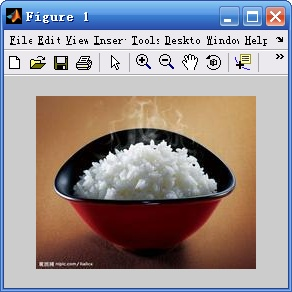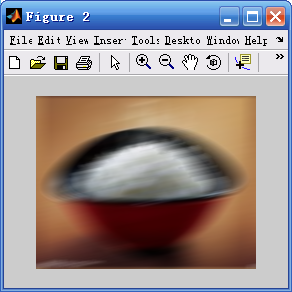原始图像 模糊化后的图像
（2）、使用deconvwnr 函数可以利用维纳滤波方法恢复图像。
I=I(10+[1:256],222+[1:256],:);
PSF=fspecial(‘motion’,LEN,THETA);
Blurred=imfilter(I,PSF,’circular’,’conv’);
wnrl=deconvwnr(Blurred,PSF);
imshow(wnrl);
结果如图所示：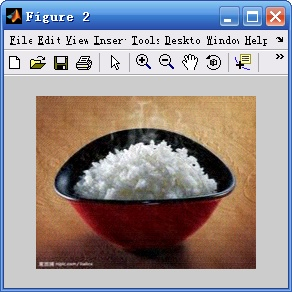2,、（1）将一幅图像读入MATLAB 工作空间。本例使用裁剪来减小要恢复的图像的大小。
I=I(10+[1:256],222:);
figure;imshow(I);
结果如图所示：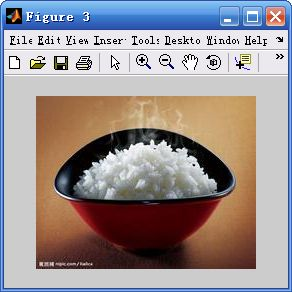（2 ）创建PSF
PSF=fspecial(‘gaussian’,11,5);
（3）模糊化图像并添加噪声。
Blurred=imfilter(I,PSF,’conv’);
V=0.02;
BlurredNoisy=imnoise(Blurred,’gaussian’,0,V);
figure;imshow(BlurredNoisy);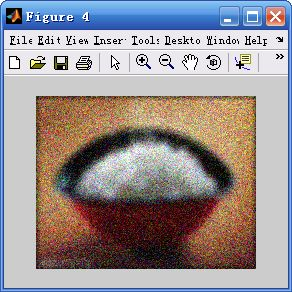（4 ）用deconvreg 函数恢复图像，指定PSF 和噪声幂次NP 。
NP=V*prod(size(I));
[reg1 LAGRA]=deconvreg(BlurredNoisy,PSF,NP);
figure,imshow(reg1);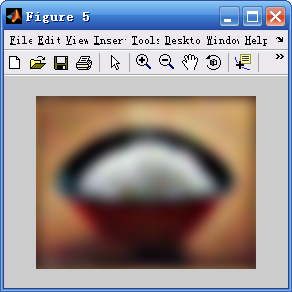展开全文算法 python 计算机视觉 opencv
• 这是我用Matlab写的，用维纳滤波处理运动模糊，请高手指点一下，希望对大家有帮助 这是我用Matlab写的，用维纳滤波处理运动模糊，请高手指点一下，希望对大家有帮助
• 基于MATLAB的医学影像图像恢复实现研究.pdf
• 江 西 理 工 大 学 本 科 毕 业 设 计 论文 题 目基于MATLAB的维纳滤波在图像恢复中的应用 1 摘 要 本文主要研究的是基于MATLAB的维纳滤波在图像恢复中的应用, 在实际的 日常生活中人们要接触很多图像画面而在景物...
•   首先举个例子说明插值过程，先看看matlab的插值函数 interp() 吧： x = -2 : 1 : 2; y = -2 : 1 : 2; [X, Y] = meshgrid(x, y); Z = -X.^2 - Y.^2; p = -2 : 0.33 : 2; q = -2 : 0.33 : 2; [P, Q] = meshgrid(p,...
• matlab几个图像恢复的程序代码-deconv_tuberlin.m 主要针对运动模糊和离焦模糊，这是我的硕士课题之一，有兴趣的一起讨论下。
• matlab如何恢复删除的代码在您的 Game Boy 相机保存中插入自定义图片，在迷你游戏中作弊并解锁 B 专辑 作者：Raphaël BOICHOT，2021 年 5 月，一个 Matlab/Octave 项目。 这个想法（再次）来自 Game Boy Camera ...
• 图像处理从内核恢复模糊图像。 “ImageRestoration.m”——在 Matlab 中实现一个 GUI，用于应用逆滤波、截断逆滤波、维纳滤波和约束最小二乘滤波“metrics.m”——一个用户定义的函数来计算 PSNR 和 SSIM。 在...
• ## MATLAB图像复原

千次阅读 2019-05-18 22:07:54
%f是一个大小为512*512的白色图像 %叠加了高斯噪声的图像，噪声均值为0,方差为0.01 g1 =imnoise( f, 'gaussian', 0, 0.01);imshow( g1, []);%显示退化的图像 %叠加了椒盐噪声的图像，１％的像素受到污染 g2 = ...
f = ones(512,512);%f是一个大小为512*512的白色图像
%叠加了高斯噪声的图像，噪声均值为0,方差为0.01
g1 =imnoise( f, 'gaussian', 0, 0.01);imshow( g1, []);%显示退化的图像

%叠加了椒盐噪声的图像，１％的像素受到污染
g2 = imnoise( f, 'salt & pepper', 0.01);

figure,imshow(g2,[]);%显示退化的图像
%叠加了乘性噪声的噪声，噪声均匀分布，均值为0，方差为0.01，g3=f+n*f
g3=imnoise(f,'speckle',0.01);
figure,imshow(g3,[]);%显示退化的图像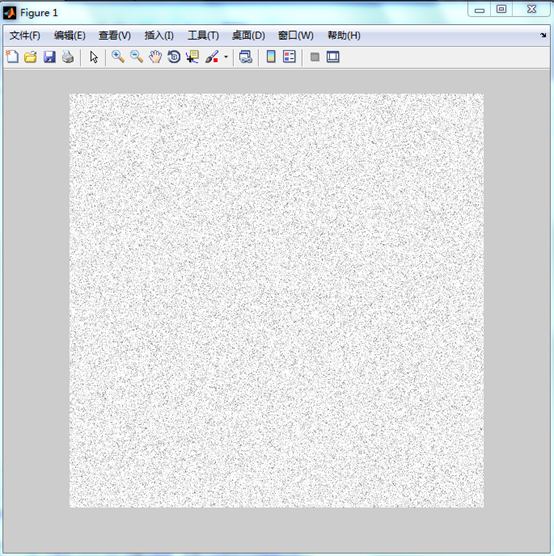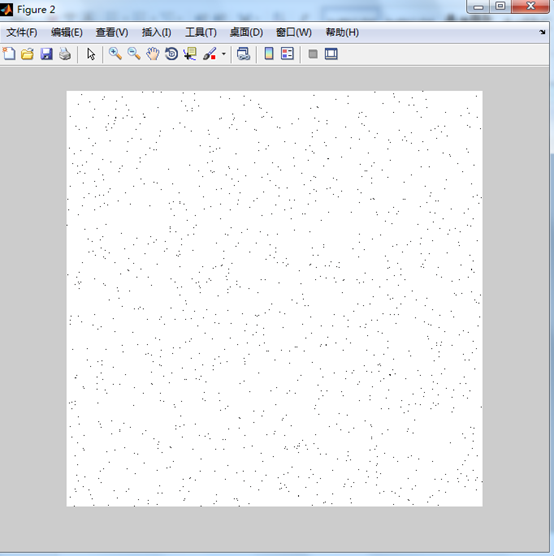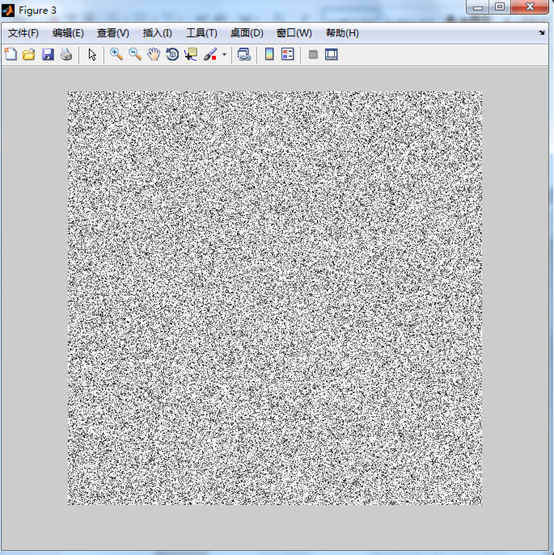f=imread('你的图');
subplot(3,3,1);imshow(f,[]);title('(f)原始图像');
[M,N]=size(f);
R=imnoise2('salt & pepper',M,N,0.1,0);        %产生胡椒噪声
c=find(R==0);                       %记录噪声对应点的坐标
gp=f;                              %复制图像
gp(c)=0;                           %将图像中胡椒对应地点的值赋为0
subplot(3,3,2);imshow(gp,[]);title('胡椒噪声')
R=imnoise2('salt & pepper',M,N,0.1,0);        %产生盐粒噪声
c=find(R==0);                       %记录噪声对应点的坐标
gs=f;                              %复制图像
gs(c)=255;                            %将图像中盐粒对应地点的值赋为0
subplot(3,3,3);imshow(gs,[]);title('盐粒噪声');
fp=spfilt(gp,'midpoint',3,3);            %使用中点平均滤波器去除胡椒噪声
subplot(3,3,4);imshow(fp,[]);title('中点滤波器');
fs=spfilt(gs,'median',3,3);              %使用中值滤波器去除胡椒噪声
subplot(3,3,5);imshow(fs,[]);title('中值滤波器');
fpmax=spfilt(gp,'max',3,3);            %使用3x3最大值滤波器去除胡椒噪声
subplot(3,3,6);imshow(fpmax,[]);title('最大值滤波器');
fsmin=spfilt(gs,'min',3,3);             %使用3x3最小值滤波器去除盐粒噪声
subplot(3,3,7);imshow(fsmin,[]);title('最小值滤波器');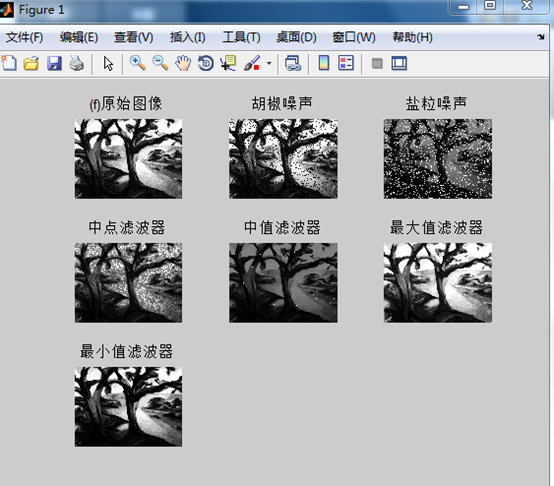f=imread('你的图');%加载测试图像，f参见图5-1
[M,N]=size(f);%M、N是测试图像的大小
C=[6 32;-2 2];%C是周期噪声在频谱上的位置
A=[10000 50000];%A是振幅向量
[r,R,S]=imnoise3(M,N,C,A);%产生周期噪声
imshow(S,[]);%S是周期噪声的频谱
figure,imshow(r,[]);%r是周期噪声图像，有明显的明暗条纹显示
g=im2double(f)+r;   %噪声图像放大10000倍后与测试图像相加
figure,imshow(g,[])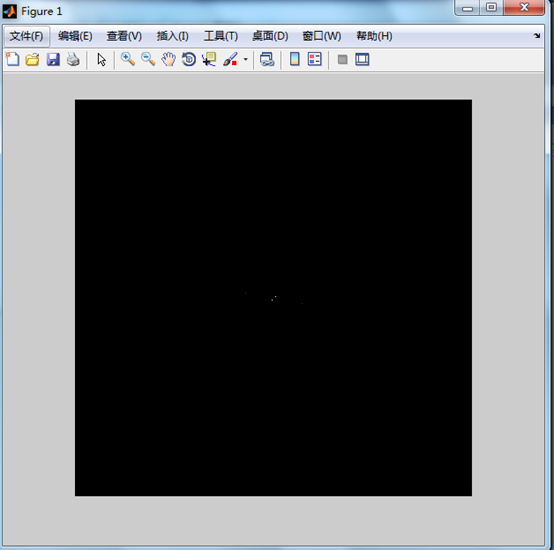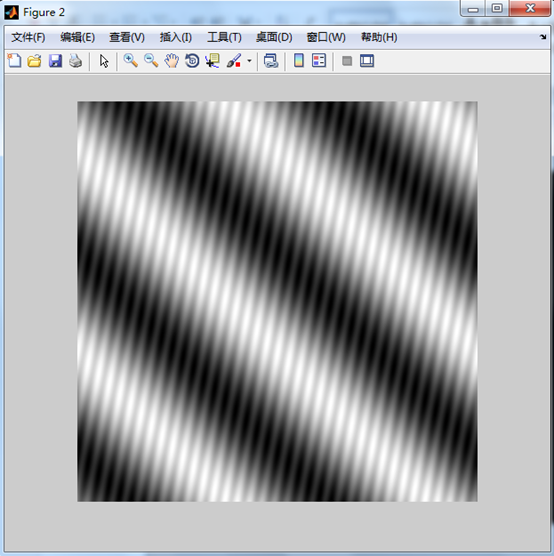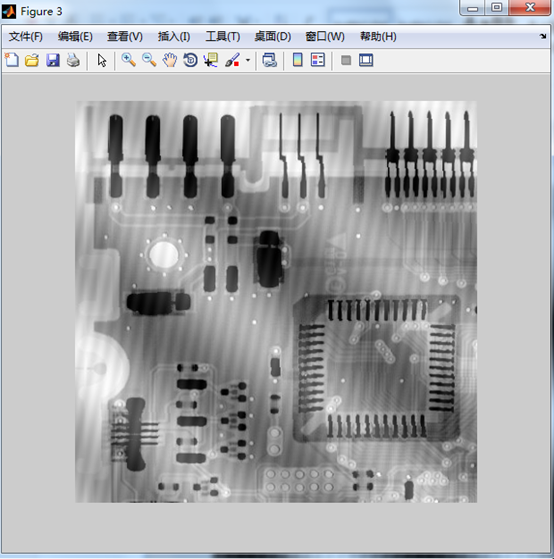f=checkerboard(8);%创建一副黑白棋盘格测试图像
PSF=fspecial('motion',7,45);%创建运动模糊退化函数
gb=imfilter(f,PSF,'circular');%产生退化图像
noise=imnoise(zeros(size(f)),'gaussian',0,0.001);
g=gb+noise;%退化图像叠加高斯噪声
imshow(g,[]);%显示退化图像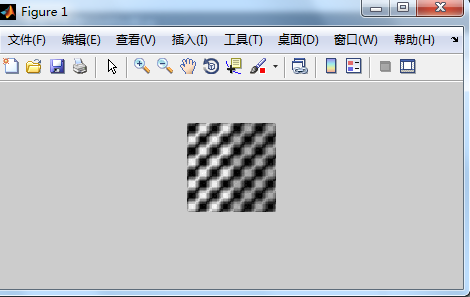f=checkerboard(8);            %创建一副黑白棋盘格测试图像
subplot(2,3,1); imshow(f,[]); title('(f)原始图像');
PSF=fspecial('motion',7,45);      %创建运动模糊退化函数
gb=imfilter(f,PSF,'circular');       %产生退化图像
g=imnoise(gb,'gaussian',0,0.01);   %退化图像叠加高斯噪声
subplot(2,3,2); imshow(g,[]); title('(g)加燥和运动模糊图像');
fr1=deconvwnr(g,PSF);           %直接逆滤波复原噪声图像
subplot(2,3,3);imshow(fr1,[]);title('(fr1)逆滤波复原图像');
Sn=abs(fft2(g)).^2;          %噪声功率谱
nA=sum(Sn( : ))/numel(g);    %噪声平均功率,此处将原来的prod(size(X))函数，变为numel(x);
Sf=abs(fft2(f)).^2;           %图像功率谱
fA=sum(Sf( : ))/numel(f);     %图像平均功率
R=nA/fA;                  %R是噪信比
fr2=deconvwnr(g,PSF,R);      %采用常系数对图像进行维纳滤波
subplot(2,3,4);imshow(fr2,[]);title('(fr2)常数比率维娜滤波复原图像');
NCORR=fftshift(real(ifft2(Sn)));        %NCORR是噪声的自相关函数
ICORR=fftshift(real(ifft2(Sf)));           %ICORR是原图像的自相关函数
fr3=deconvwnr(g,PSF,NCORR,ICORR);     %使用自相关函数的滤波方法
subplot(2,3,5);imshow(fr3,[]);title('(fr3)自相关函数维娜滤波复原图像');
fr4=deconvreg(g,PSF,0.4,[1e-7,1e7]);     %使用约束的最小二乘方滤波
subplot(2,3,6);imshow(fr4,[]);title('(fr4)最小二乘方滤波复原图像');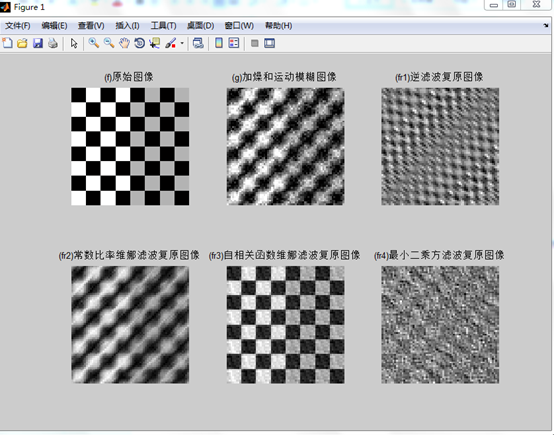fr1=deconvwnr(g,PSF);%直接逆滤波复原噪声图像
figure,imshow(fr1,[]);

Sn=abs(fft2(noise)).^2;%噪声功率谱
nA=sum(Sn( : ))/prod(size(noise));%噪声平均功率
Sf=abs(fft2(f)).^2;%图像功率谱
fA=sum(Sf( : ))/prod(size(f));%图像平均功率
R=nA/fA;%R是噪信比
fr2=deconvwnr(g,PSF,R);%采用常系数对图像进行维纳滤波
figure,imshow(fr2,[]);

NOCRR=fftshift(real(ifft2(Sn)));%NCORR是噪声的自相关函数
ICORR=fftshift(real(ifft2(Sf)));%ICORR是原图像的自相关函数
fr3=deconvwnr(g,PSF,NCORR,ICORR);%使用自相关函数的滤波方法
figure,imshow(fr3,[]);

fr4=deconvreg(g,PSF,0.4,[1e-7,1e7]);%使用约束的最小二乘方滤波
figure,imshow(fr4,[]);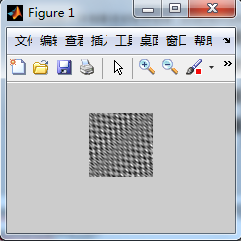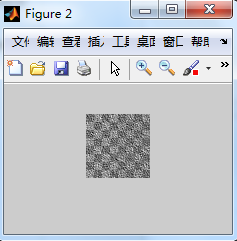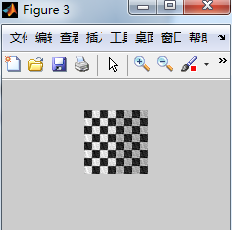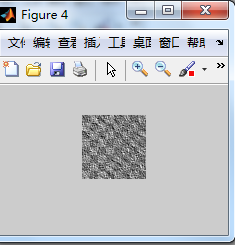f=checkerboard(50);%产生测试棋盘格图像
s=0.8; %s是缩放稀疏
theta=pi/6;%theta是旋转角度
%T是仿射变换矩阵
T=[s*cos(theta) s * sin(theta) 0; -s * sin(theta) s * cos(theta) 0; 0 0 1];
tform=maketform('affine',T);       %thorm是仿射变换结构体
g=imtransform(f,tform);           %对图像f施加仿射变换
imshow(f);
figure,imshow(g,[]);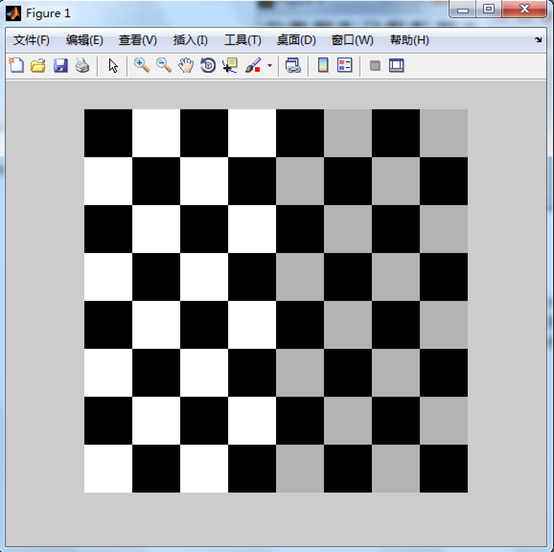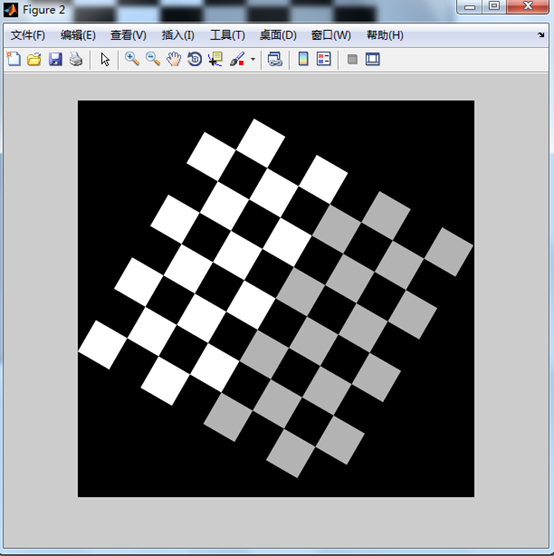展开全文• matlab盲卷积算法和Lucy迭代算法对模糊噪声图像恢复
• 图像在采集、传送、转换过程中会混入噪声，造成图像模糊、失真、有噪声，图像复原技术的目的是使图像尽可能恢复到原本的样子。 一、常见的噪声类型 高斯噪声 椒盐噪声 均匀分布噪声 指数分布噪声 伽马分布噪声 在...


文章目录
图像复原技术一、常见的噪声类型二、空域内的滤波复原方法1、均值滤波(1)、算术均值滤波(2)、几何均值滤波(3)、谐波均值滤波器(4)、逆谐波均值滤波器
2、统计排序滤波(1)、中值滤波器(2)、最大值滤波器(3)、最小值滤波器(4)、中点滤波器
3、自适应滤波
三、图像复原方法1、逆滤波

完整目录

图像复原技术
图像在采集、传送、转换过程中会混入噪声，造成图像模糊、失真、有噪声，图像复原技术的目的是使图像尽可能恢复到原本的样子。
一、常见的噪声类型
高斯噪声椒盐噪声均匀分布噪声指数分布噪声伽马分布噪声
在Matlab中，函数 imnoise 可以给图像加入高斯噪声、椒盐噪声、泊松分布噪声和乘性噪声
二、空域内的滤波复原方法
1、均值滤波
(1)、算术均值滤波
令 Sxy 表示中心在点(x, y)处、大小为 m * n的矩形子窗口区域(邻域)的一组坐标，然后计算这一组坐标所在像素值的平均值，将其赋值给点 (x, y)。
通俗点讲，就是选图像上的一块区域，把这块区域的所有像素点相加求平均值再赋值给这块区域中心的那个像素点，后边的几种方法只是算法不同而已。
公式为：

f

^

(

x

,

y

)

=

1

m

n

∑

(

s

,

t

)

∈

S

x

y

g

(

s

,

t

)

\hat{f}(x, y) = \frac{1}{mn}\sum_{(s, t) \in S_{xy}}g(s, t)

(2)、几何均值滤波
算术均值滤波是把 Sxy 所代表点的像素值求和再取平均值，而几何均值滤波是把像素值求乘再求像素的数量次幂。几何均值滤波实现的平滑可与算术均值滤波相比，且丢失的图像细节更少 公式为：

f

^

(

x

,

y

)

=

[

∏

(

s

,

t

)

∈

S

x

y

g

(

s

,

t

)

]

1

m

n

\hat{f}(x, y) = \left[ \prod_{(s, t) \in S_{xy}}g(s, t)\right]^{\frac{1}{mn}}

(3)、谐波均值滤波器
谐波均值滤波器对于盐粒噪声效果很好，但不适用于胡椒噪声，擅于处理高斯噪声 公式为：

f

^

(

x

,

y

)

=

m

n

∑

(

s

,

t

)

∈

S

x

y

1

g

(

s

,

t

)

\hat{f}(x, y) = \frac{mn}{\sum_{(s, t) \in S_{xy}} \frac{1}{g(s, t)}}

(4)、逆谐波均值滤波器
Q 称为滤波器的阶数，适用于减少或在实际中消除椒盐噪声干扰的影响。当 Q 为正时，该滤波器可消除胡椒噪声，当 Q 为负值时该滤波器可消除盐粒噪声，当 Q 为 0 时，公式可化简为算术均值滤波，当 Q = -1 时则为谐波均值滤波器。 公式为：

f

^

(

x

,

y

)

=

∑

(

s

,

t

)

∈

S

x

y

g

(

s

,

t

)

Q

+

1

∑

(

s

,

t

)

∈

S

x

y

g

(

s

,

t

)

Q

\hat{f}(x, y) = \frac{\sum_{(s, t) \in S_{xy}}g(s, t)^{Q+1}}{\sum_{(s, t) \in S_{xy}}g(s, t)^{Q}}

下列代码为算术均值滤波和几何均值滤波的实例代码及结果：
I = imread('火影9.jpg');
I = im2double(I);
I = imnoise(I, 'gaussian', 0.05);   %加入高斯噪声
%算术均值滤波
PSF = fspecial('average', 3);   %创建预定义的二维滤波器
J = imfilter(I, PSF);
%几何均值滤波
K = exp(imfilter(log(I), PSF));

figure;
subplot(131);imshow(I);title('加入高斯噪声');
subplot(132);imshow(J);title('算术均值滤波');
subplot(133);imshow(K);title('几何均值滤波');

冈萨雷斯大佬的《数字图像处理》那本书上确实写了几何均值滤波比算术均值滤波要保留更多细节，我tm咋感觉是假的呢 o(一︿一+)o，结果如下：2、统计排序滤波
其实我觉得和均值滤波的原理差不多，都是选取一块区域，把这块区域里的像素们(一长串数字)进行各种运算操作。
(1)、中值滤波器
中值滤波函数：medfilt2
正如其名字一样，使用一个像素邻域中的灰度级的中值来替代该像素的值。该滤波器针对单极或双极脉冲噪声尤其有效 公式为：

f

^

(

x

,

y

)

=

m

e

d

i

a

n

(

s

,

t

)

∈

S

x

y

{

g

(

s

,

t

)

}

\hat{f}(x, y) = median_{(s, t) \in S_{xy}} \left\{ g(s, t) \right\}

I = imread('火影9.jpg');
I = im2double(I);
I = imnoise(I, 'salt & pepper', 0.05);   %加入椒盐噪声

J = medfilt2(I, [3, 3]);    %中值滤波

figure;
subplot(121);imshow(I);title('加入椒盐噪声');
subplot(122);imshow(J);title('中值滤波');

从结果可以看出，图像很好的去除了椒盐噪声，但是图像有些模糊了：(2)、最大值滤波器
就是对邻域中的所有像素点取最大值赋值给该像素点，最大值滤波器对于发现图像中的最亮点非常有用！另外它会从黑色物体边缘去除一些黑色像素 公式为：

f

^

(

x

,

y

)

=

m

a

x

(

s

,

t

)

∈

S

x

y

{

g

(

s

,

t

)

}

\hat{f}(x, y) = max_{(s, t) \in S_{xy}} \left\{ g(s, t) \right\}

(3)、最小值滤波器
就是对邻域中的所有像素点取最小值赋值给该像素点，最小值滤波器对于发现图像中的最暗点非常有用！另外它会从白色物体边缘去除一些白色像素 公式为：

f

^

(

x

,

y

)

=

m

i

n

(

s

,

t

)

∈

S

x

y

{

g

(

s

,

t

)

}

\hat{f}(x, y) = min_{(s, t) \in S_{xy}} \left\{ g(s, t) \right\}

(4)、中点滤波器
选取最大值与最小值的中点(就是最大最小值的平均)替换该点的像素值，结合了统计排序和求平均，对于处理随机噪声表现很好，比如高斯噪声或均匀噪声。 公式为：

f

^

(

x

,

y

)

=

1

2

[

m

a

x

(

s

,

t

)

∈

S

x

y

{

g

(

s

,

t

)

}

+

m

i

n

(

s

,

t

)

∈

S

x

y

{

g

(

s

,

t

)

}

]

\hat{f}(x, y) = \frac{1}{2} [ max_{(s, t) \in S_{xy}} \left\{ g(s, t) \right\} + min_{(s, t) \in S_{xy}} \left\{ g(s, t) \right\} ]

二维顺序统计滤波函数：ordfilt2，可以实现中值、最大最小值滤波
I = imread('火影9.jpg');
I = rgb2gray(I);
I = im2double(I);
I = imnoise(I, 'salt & pepper', 0.1);   %加入椒盐噪声

domain = [1 1 1 1; 1 1 1 1; 1 1 1 1; 1 1 1 1];
J1 = ordfilt2(I, 1, domain);
J2 = ordfilt2(I, 8, domain);
J3 = ordfilt2(I, 16, domain);

figure;
subplot(221);imshow(I);title('加入椒盐噪声');
subplot(222);imshow(J1);title('最小值滤波');
subplot(223);imshow(J2);title('中值滤波');
subplot(224);imshow(J3);title('最大值滤波');

结果展示：3、自适应滤波
二维自适用去噪滤波：wiener2，自适应滤波器的性能比目前所有滤波器的性能都好
I = imread('火影9.jpg');
I = rgb2gray(I);

%I = imcrop(I, [100, 100, 200, 200]);    %图像裁剪

I = imnoise(I, 'gaussian', 0, 0.003);   %加入高斯噪声
[K, noise] = wiener2(I, [5, 5]);

figure;
subplot(121);imshow(I);title('加入高斯噪声');
subplot(122);imshow(K);title('自适应滤波');

可以看出，效果还不错：三、图像复原方法
1、逆滤波
大意就是我们知道了原图受到了什么算法(叫做退化函数 H )带来的噪声，那么我们从噪声图反着用这个算法就可以推算出原图。 不过即使知道了退化函数，也还是不能完全恢复到原图。
I = imread('cameraman.tif');
I = im2double(I);
[m, n] = size(I);
M = 2 * m;
N = 2 * n;

u = -m / 2:(m / 2 - 1);
v = -n / 2:(n / 2 - 1);
[U, V] = meshgrid(u, v);           %基于向量 u 和 v 中包含的坐标返回二维网格坐标
D = sqrt(U.^2 + V.^2);
D0 = 130;                           %滤波器截止频率为20
H = exp(-(D.^2)./(2 * (D0^2)));
N = 0.01 * ones(size(I, 1), size(I, 2));
N = imnoise(N, 'gaussian', 0, 0.001);
J = fftfilter(I, H) + N;

HC = zeros(m, n);
M1 = H > 0.1;
HC(M1) = 1 ./ H(M1);
K = fftfilter(J, HC);
HC = zeros(m, n);
M2 = H > 0.01;
HC(M2) = 1 ./ H(M2);
L = fftfilter(J, HC);

figure;
subplot(221);imshow(J);
subplot(222);imshow(J, []);
subplot(223);imshow(K, []);
subplot(224);imshow(L, []);

其中，fftfilter函数如下：
function Z = fftfilter(X, H)
F = fft2(X, size(H, 1), size(H, 2));
Z = H .* F;
Z = ifftshift(Z);
Z = abs(ifft2(Z));
Z = Z(1:size(X, 1), 1:size(X, 2));
end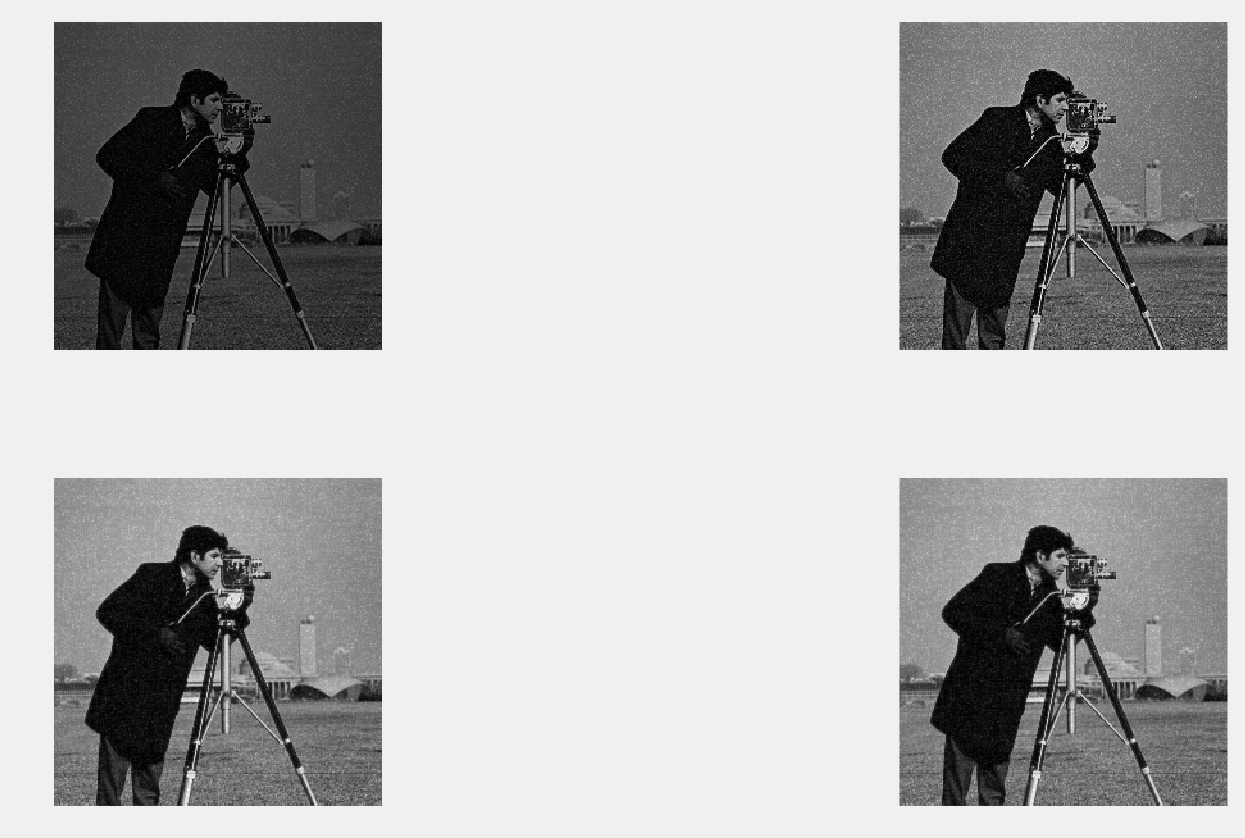完整目录
Matlab数字图像处理——图像处理工具箱Image Processing Toolbox Matlab数字图像处理——图像类型的转换 Matlab数字图像处理——图像文件的读取 Matlab数字图像处理——图像文件的显示 Matlab数字图像处理——视频文件的读写 Matlab数字图像处理——图像的像素运算(灰度变换) Matlab数字图像处理——图像的空间变换 Matlab数字图像处理——图像的平移、邻域操作、区域选取 Matlab数字图像处理——图像增强 Matlab数字图像处理——图像复原
展开全文• matlab几个图像恢复的程序代码-deconv_sondhi.m 主要针对运动模糊和离焦模糊，这是我的硕士课题之一，有兴趣的一起讨论下。
• 数字图像水印中使用Arnold置乱，可以起到加密和保护的作用，此代码就是对原始图像进行置乱，然后对置乱后的图像进行恢复的实现，代码附有详细的注释说明，简单易懂。
• matlab图像处理_matlab图片水印_matlab图像加密_加密水印_图像水印_bd486187_源码.zip
• 设计目标及需求分析 设计目标：希望通过matlab设计一个软件来实现对CT图像的模糊再恢复的过程，是对现实中CT图像复原的一个简单仿真。需求分析：随着网络和通信技术的发展，数字图像处理与分析技术已经在科学研究、...python java 机器学习 计算机视觉 编程语言
• matlab图像进行Lucy-Richardson滤波恢复，包含代码的实现
• 恢复极度模糊或退化的图像。 由于许多因素，例如相机和行驶中的汽车之间的相对运动（例如超速行驶的汽车的图像），图像变得模糊。 逆滤波器、维纳滤波器和露西-理查森滤波器用于恢复图像。 光流分析，即模糊长度和...
• 其按照特定的需要突出一幅图像中的某些信息或强化某些感兴趣的特征，将原来不清晰的图片变得清晰，使之改善图像质量和丰富信息量，提高图像的视觉效果和图像成分的清晰度，加强图像判读和识别效果的图像处理的方法。...
• 数字图像处理课程第六次作业的代码，包括高斯噪声、椒盐噪声、高斯滤波、中值滤波、反谐波均值滤波、运动模糊、维纳滤波、约束最小二乘滤波。参考课本是冈萨雷斯《数字图像处理》英文第三版
• 这是对MATLAB函数中图像恢复函数的讲解，希望对大家有所帮助！
• 算法图像恢复代码matlab 帮助说明 GPUhelp文件说明了GPU的连接和使用方法。 论文参考 包含参考过的论文原文，论文复现记录。 env是本地virtualenv环境。 pytorch笔记是从pytorch官方文档里摘录的参考。 项目进度书 ...
• 图像的恢复实验目的实验环境相关知识1.imhist2. imnoise3. getrect4. imcrop5. std2 计算图像的标准差6．信噪比的计算方法：图像的信噪比应该等于信号与噪声的功率谱之比...1、熟悉并掌握MATLAB图像处理工具箱的使用；
• matlab函数用于图像边缘的提取，包含的方法有说soble算子...

# matlab图像恢复matlab 订阅